# Retrieving the Minimal / Maximal Values from a Filtered List in Microsoft Excel 2010

In this article, we will learn how to retrieve the minimal or maximal values from a filtered list. We will use the Subtotal function in Microsoft Excel 2010 to derive the desired output.

SUBTOTAL: - This function is used for returning a subtotal in a list or database. By using this function, we can return the Average, Count etc.

The Syntax of SUBTOTAL function:         =SUBTOTAL(Function_num,ref1,….)

For example: We have a list in column A. If we want to return the average of the numbers, we need to follow the below given steps.

• Write the formula in cell B2.
• =SUBTOTAL(1,A2:A8)
• Press Enter on your keyboard.
• The function will return the average value of list 1.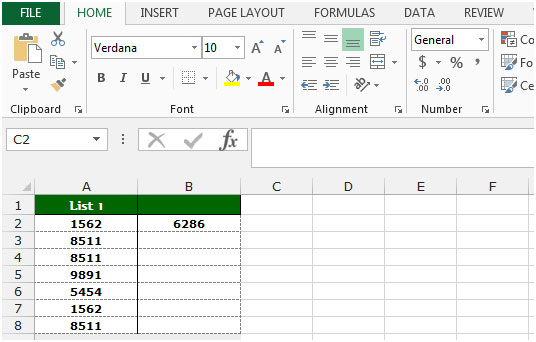Let’s take an example to understand how we can retrieve the minimal and maximal values from a filtered list:-

We have data in range A1:C10 in which column A contains country name, column B contains Agent name, and column c contains sales amount.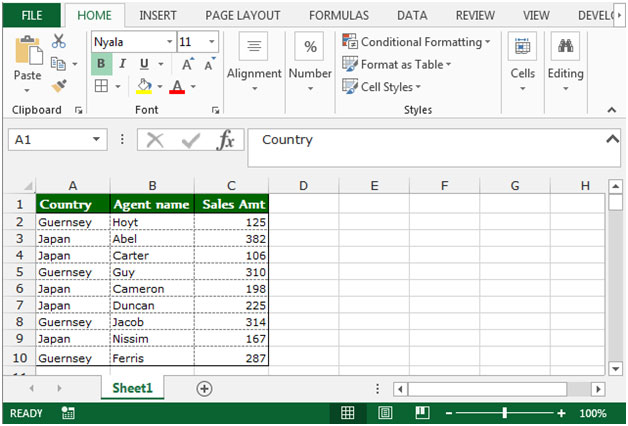Now, we want to put the filter in column A (country), and then want to return the minimal and maximal values from the filtered list.

• Select the data and then go to Data tab in ribbon.
• Click on Filter from the Sort & Filter group.
• Click on the country filter, and select Guernsey.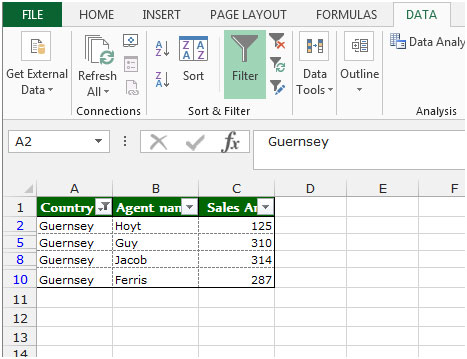• Write the formula in cell D2.
• =SUBTOTAL(5,C2:C10)
• Press Enter on your keyboard.
• The function will return the minimal value from the filtered data.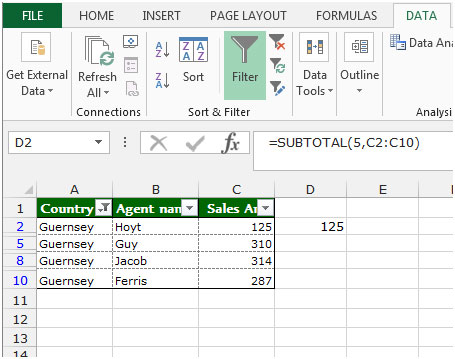To return the maximal value, follow below given steps:-

• Write the formula in cell E2.
• =SUBTOTAL(4,C2:C10)
• Press Enter on your keyboard.
• The function will return the maximal value from the filtered data.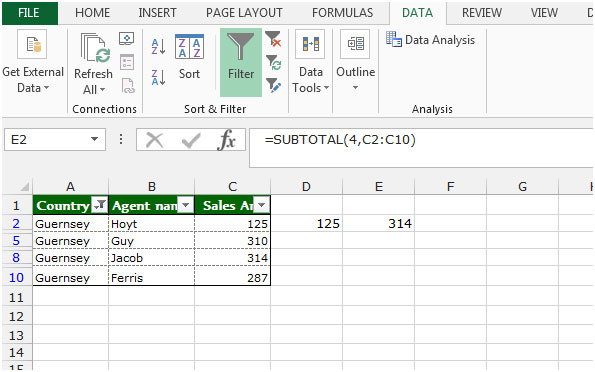Note: By using this formula, we can return the minimum and maximum value in the filtered data.

This is the way we use SUBTOTAL function in Microsoft Excel 2010.

1.